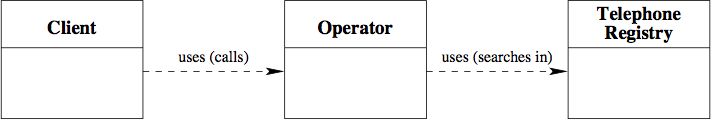Next: Realization of operations: algorithms Up: Unit 01 Previous: Representation of the domain:

## Class diagram

To make the classes and the relationships between the classes explicit, we can use the class diagram:

• each class is denoted by a rectangle containing the class name;
• the relations between classes are denoted by arrows; such arrows represent relations between classes in a simplified form, as generic usage relations;
• the properties of classes are not shown.

Example: class diagram of the CallCenter application.Class diagrams are commonly used in software design. For example, the Unified Modeling Language (UML), which is the de facto standard formalisms for software design, allows one to develop quite sophisticated class diagrams.Next: Realization of operations: algorithms Up: Unit 01 Previous: Representation of the domain: